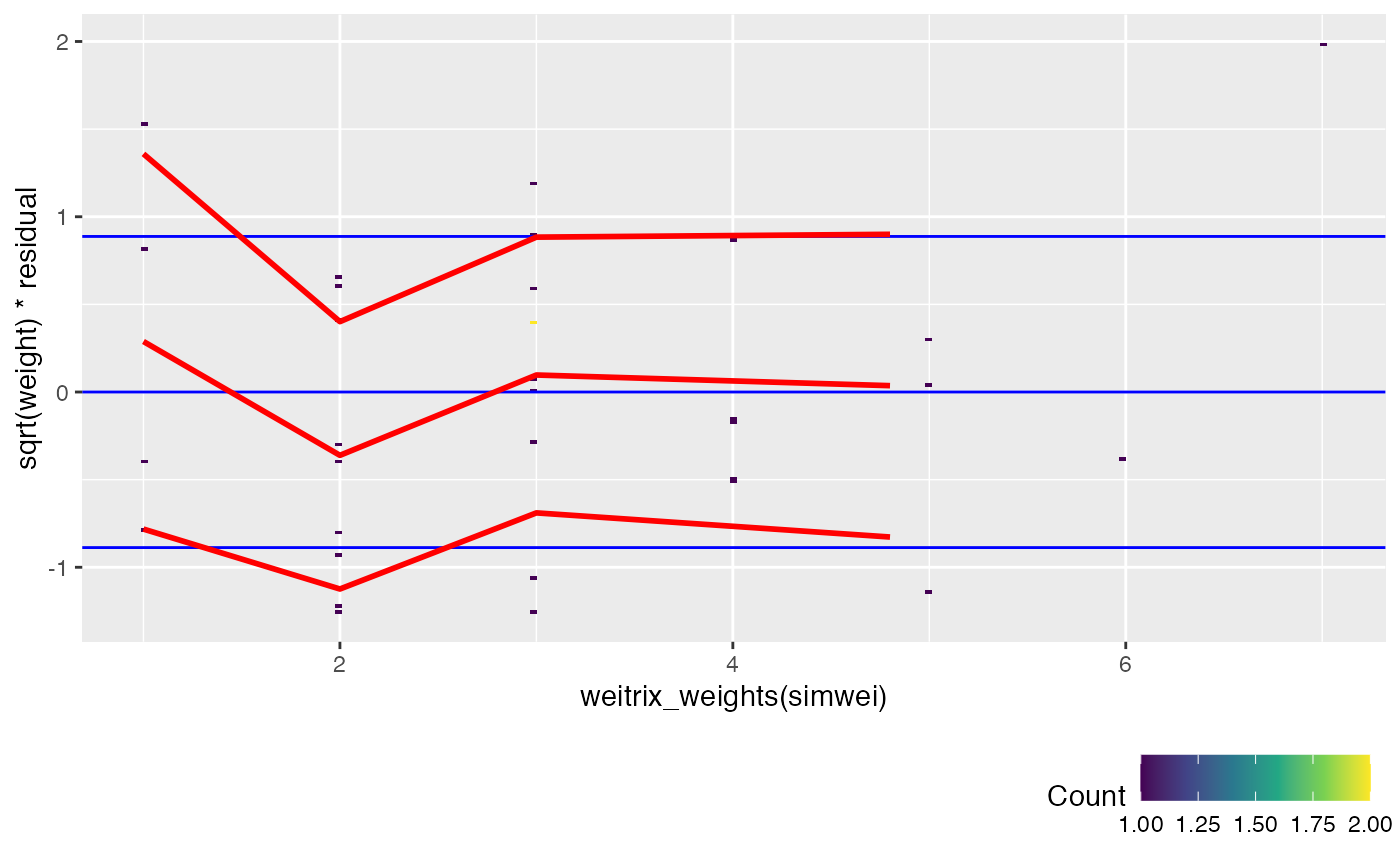Various plots based on weighted squared residuals of each element in the weitrix. weight*residual^2 is the Pearson residual for a gamma GLM plus one, as used by weitrix_calibrate_all.

weitrix_calplot(
weitrix,
design = ~1,
covar,
cat,
funnel = FALSE,
guides = TRUE
)

## Arguments

weitrix A weitrix object, or an object that can be converted to a weitrix with as_weitrix. A formula in terms of colData(weitrix or a design matrix, which will be fitted to the weitrix on each row. Can also be a Components object. Optional. A covariate. Specify as you would with ggplot2::aes. Can be a matrix of the same size as weitrix. Optional. A categorical variable to break down the data by. Specify as you would with ggplot2::aes. Flag. Produce a funnel plot? Note: covar can not be used for funnel plots. Show blue guide lines.

A ggplot2 plot.

## Details

This function is not memory efficient. It is suitable for typical bulk data, but generally not not for single-cell.

Defaults to a boxplot of sqrt(weight) weighted residuals. Blue guide bars are shown for the expected quartiles, these will ideally line up with the boxplot.

If cat is given, it will be used to break the elements down into categories.

If covar is given, sqrt(weight) weighted residuals are plotted versus the covariate, with red trend lines for the mean and for the mean +/- one standard deviation. If the weitrix is calibrated, the trend lines should be horizontal lines with y intercept close to -1, 0 and 1. Blue guide lines are shown for this ideal outcome.

Any of the variables available with weitrix_calibrate_all can be used for covar or cat.

## Examples

weitrix_calplot(simwei, ~1)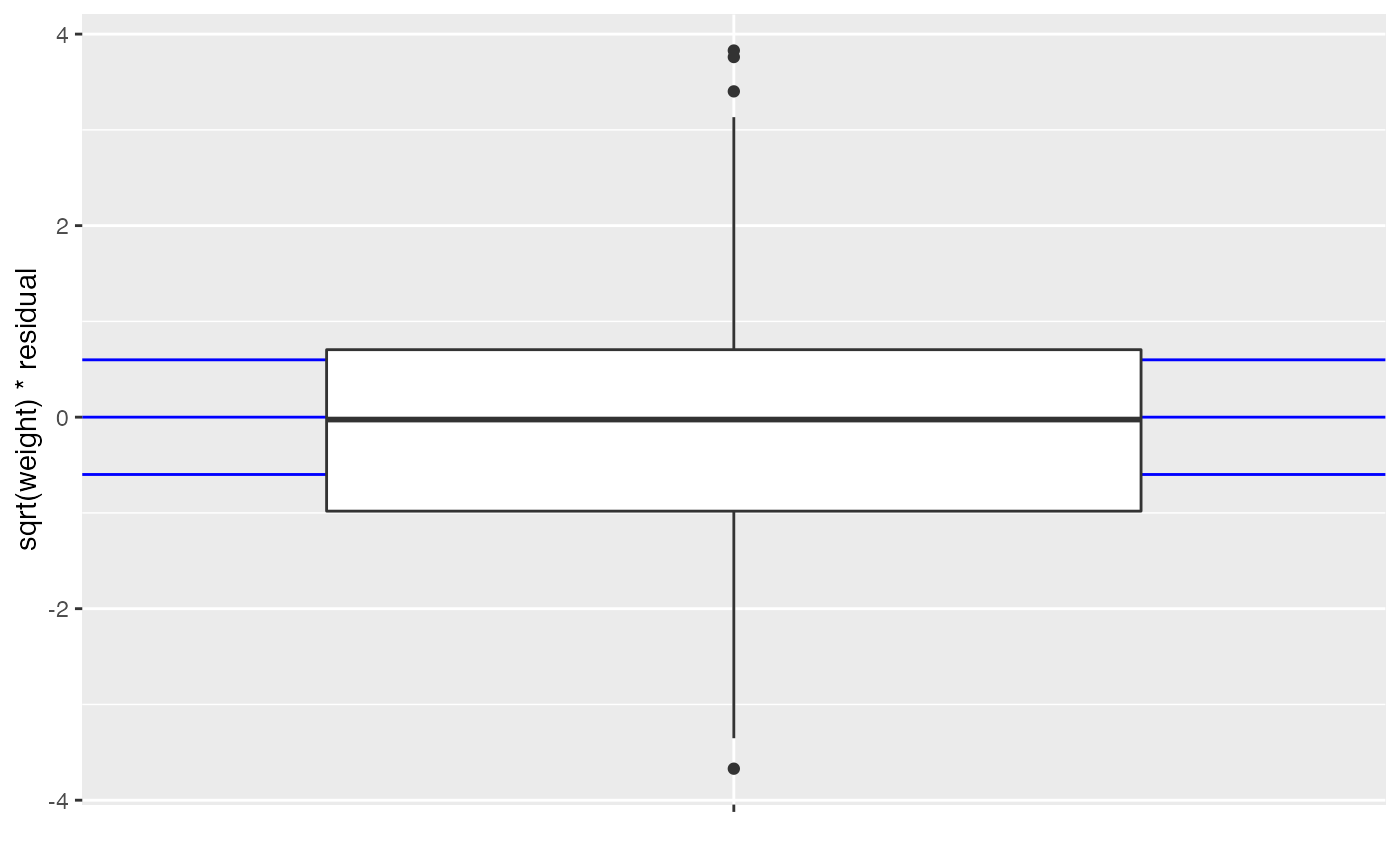weitrix_calplot(simwei, ~1, covar=mu)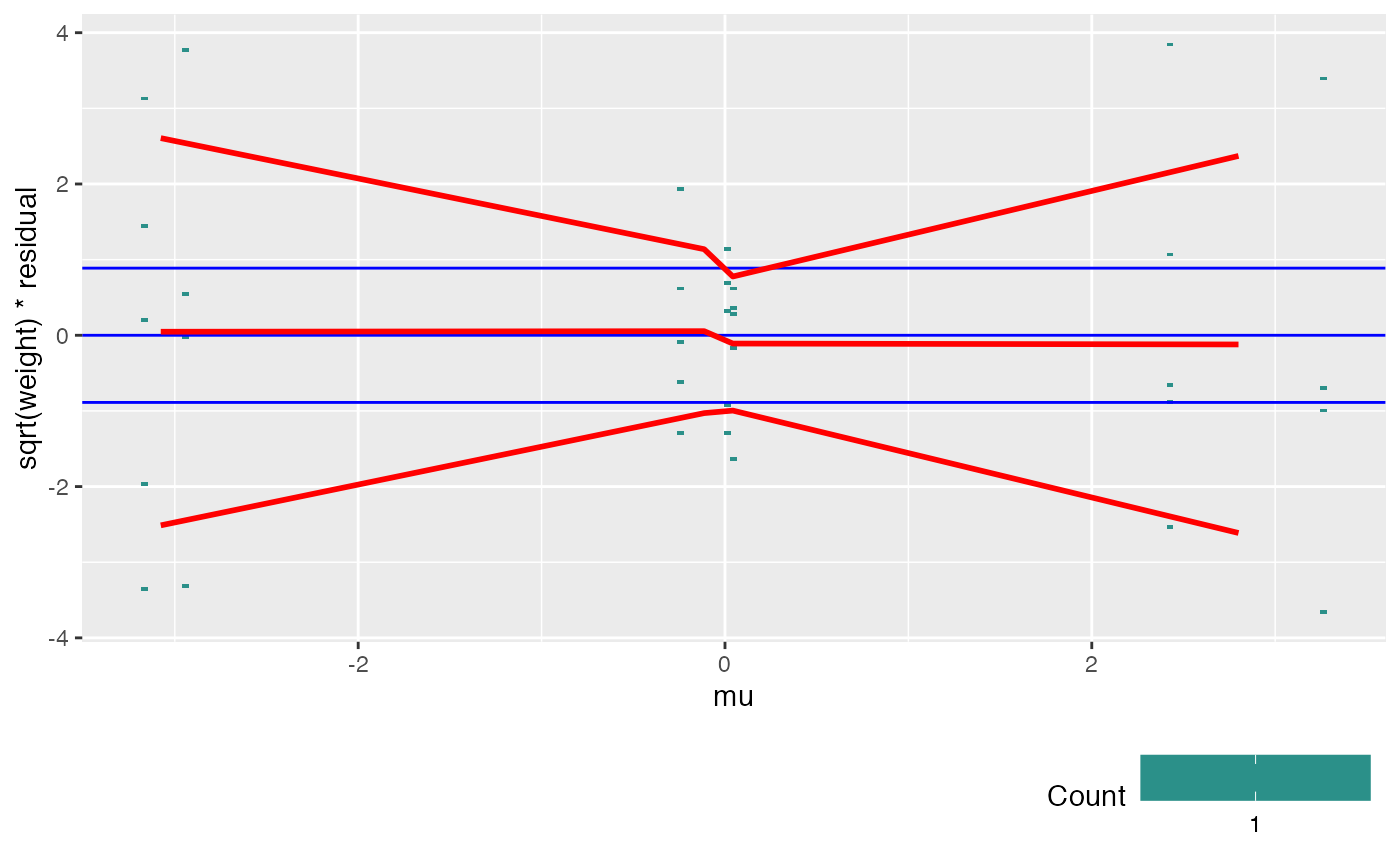weitrix_calplot(simwei, ~1, cat=col)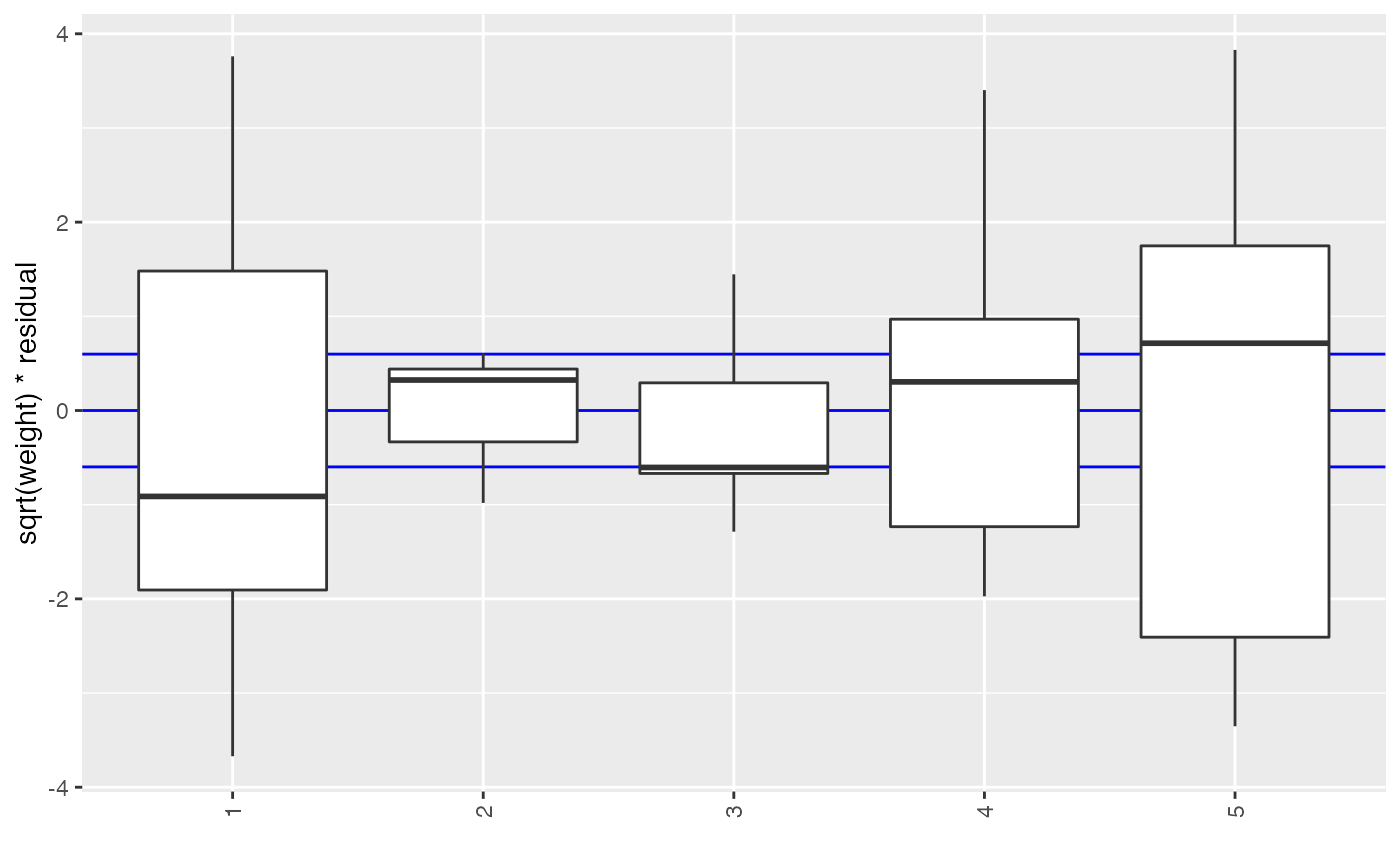# weitrix_calplot should generally be used after calibration
cal <- weitrix_calibrate_all(simwei, ~1, ~col+log(weight))
weitrix_calplot(cal, ~1, cat=col)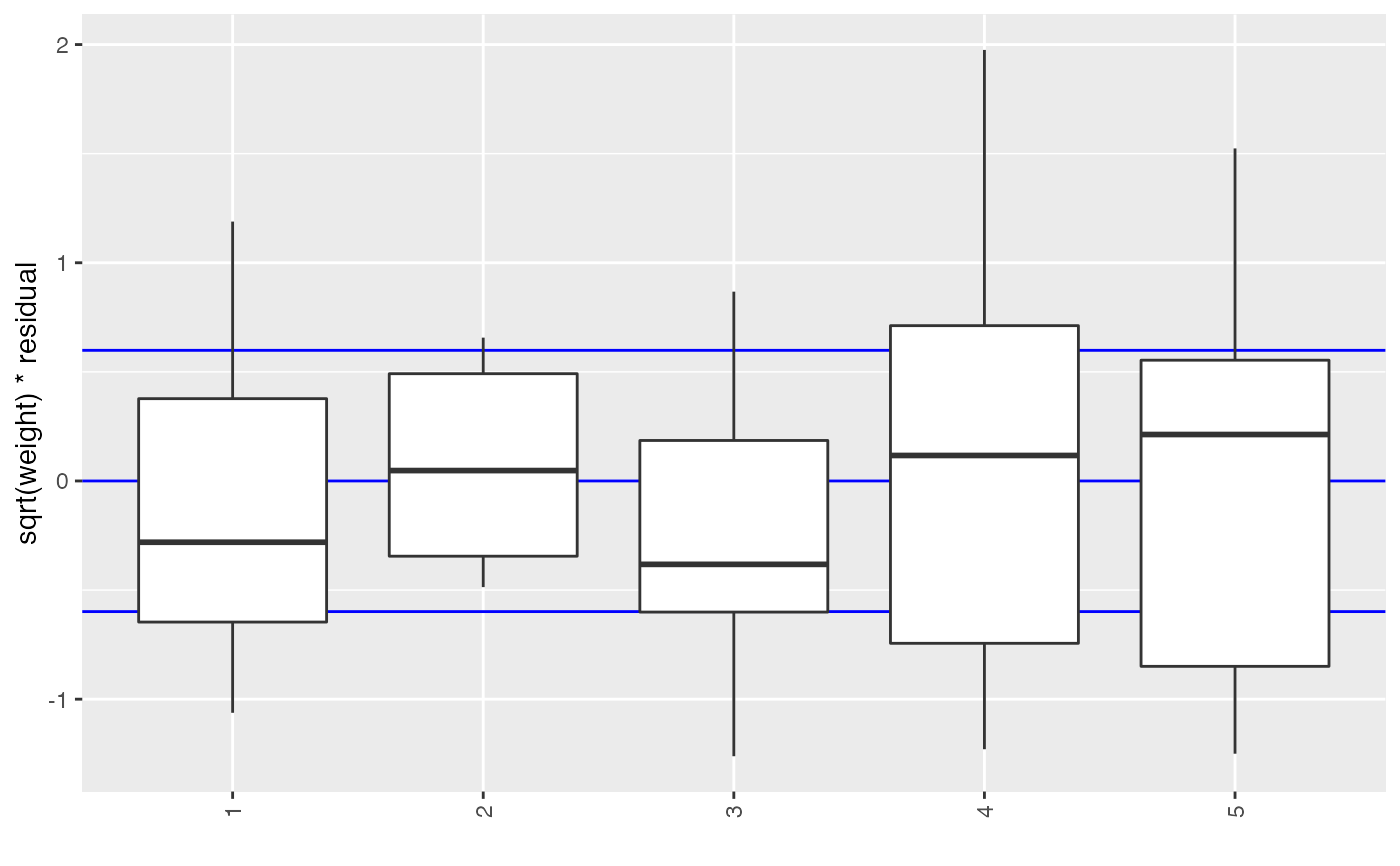# You can use a matrix of the same size as the weitrix as a covariate.
# It will often be useful to assess vs the original weighting.
weitrix_calplot(cal, ~1, covar=weitrix_weights(simwei))